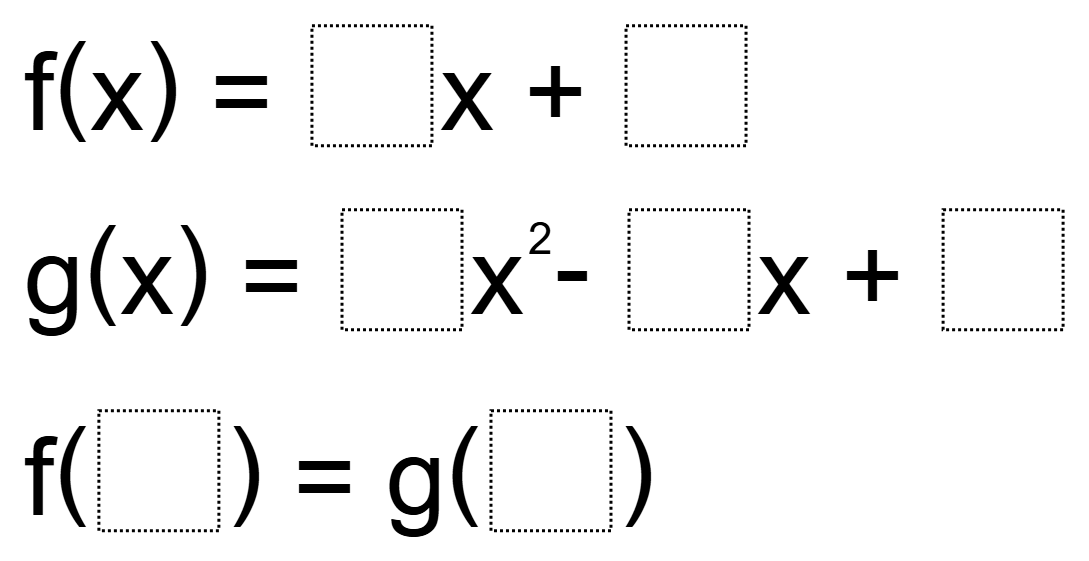Home > High School: Functions > Function Notation

# Function Notation

Directions: Using the digits 1 to 9, at most one time each, fill in the boxes so that the two functions are equivalent### Hint

How do you use function notation to determine the input and output of a function?

### Answer

One possible answer is:
f(x) = 9x + 4
g(x) = 7x^2 – 1x + 5
f(3) = g(2)

Post any others in the comments.

Source: Steven Midzak

## Creating Sequences

Directions: Using the digits 0-9, at most one time each, complete the first three terms …

### 8 comments

1.I think the format is confusing, and the answer uses incorrect notation and the two functions are, in fact, never “equivalent”. Rephrase like this?

Fill in spaces with whole numbers 1 – 9 only one time, so that the final equality is correct.
f(x) = ___ x + _____
g(x) = ___ x^2 – ____x + _____
f(____) = g(_____)

•I agree. I like the format you used better Tim.

•Thank you for this suggestion. I’ve edited the question accordingly.

2.f(3) = 2x + 4
g(1) = 7x^2 – 6x + 9

3.F(3)=9x+4 g(2)=7x^2-1x+5

4.5.Katy Howell Escobar

𝑓(3)=7𝑥+6 𝑔(2)=5𝑥^2−1𝑥+9

𝑓(8)=6𝑥+4 𝑔(5)=2𝑥^2−1𝑥+7

6.I gave this to my IM3 classes today, here are some they came up with that are NOT listed above:

f(3) = 6x + 1 g(4) = 2x^2 – 5x + 7
f(8) = 2x + 3 g(6) = 1x^2 – 4x + 7
f(1) = 3x + 6 g(2) = 4x^2 – 8x + 9
f(2) = 3x + 4 g(1) = 7x^2 – 5x + 8
f(2) = 3x + 7 g(5) = 1x^2 – 4x + 8